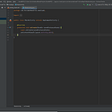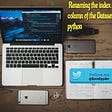# Input

• The first line contains an integer T, the number of test cases. Then the test cases follow.
• Each test case contains a single line of input, four integers D,d,P,Q.

• 1≤T≤10⁵
• 1≤d≤D≤10⁶
• 1≤P,Q≤10⁶

# Sample Input

`32 1 1 13 2 1 15 2 1 2`

`3413`

# Explanation

• On the first day, the rate of production is 1 dollar per day so 1 dollar is printed on the first day.
• On the second day, the rate of production is 1+1=2 dollars per day so 2 dollars are printed on the second day.
• The total amount of money printed in 2 days is 1+2=3 dollars.
• For the first two days, the rate of production is 1 dollar per day so 1⋅2=2 dollars are printed on the first two days.
• On the third day, the rate of production is 1+1=2 dollars per day so 2 dollars are printed on the third day.
• The total amount of money printed in 3 days is 2+2=4 dollars.
• For the first two days, the rate of production is 1 dollar per day so 1⋅2=2 dollars are printed on the first two days.
• On the next two days, the rate of production is 1+2=3 dollars per day so 3⋅2=6 dollars are printed on the next two days.
• On the last day, the rate of production is 3+2=5 dollars per day so 5 dollars are printed on the last day.
• The total amount of money printed in 5 days is 2+6+5=13 dollars.
`import java.util.*;import java.lang.*;import java.io.*;/* Name of the class has to be "Main" only if the class is public. */class Codechef{    public static void main (String[] args) throws java.lang.Exception    {        //SOLUTION        FastReader sc = new FastReader();        long t = sc.nextLong();        while(t-- > 0){        long D,d,p,q;        D = sc.nextLong();        d = sc.nextLong();        p = sc.nextLong();        q = sc.nextLong();        long n = D/d;        long sol=0;        sol= n*p*d + q*d*(n*(n-1))/2 + (D%d)*(p+n*q);        System.out.println(sol);        }    }    // Below are just the classes needed for fast Input in Java with Fast Reader    static class FastReader {        BufferedReader br;        StringTokenizer st;        public FastReader() {            br = new BufferedReader(new InputStreamReader(System.in));        }        String next() {            while (st == null || !st.hasMoreElements()) {                try {                    st = new StringTokenizer(br.readLine());                } catch (IOException e) {                    e.printStackTrace();                }            }            return st.nextToken();        }        int nextInt() {            return Integer.parseInt(next());        }        long nextLong() {            return Long.parseLong(next());        }        double nextDouble() {            return Double.parseDouble(next());        }        String nextLine() {            String str = "";            try {                str = br.readLine();            } catch (IOException e) {                e.printStackTrace();            }            return str;        }    }}`

--

--

--

## More from Anubhav Mishra

Software Developer ,currently pursuing B.Tech in Information Technology. Electronic Dance Music is love.Also like all phone related technologies

Love podcasts or audiobooks? Learn on the go with our new app.

## How to reduce APK size from 2 MB to 10 KB?## CS373 Spring 2020: Kevin Nguyen## Serverless Diary: How to Triumph Over Challenges- A2A/B2B Integration## All Things Rust## 5 Tactics Agency Recruiters use to hinder your job search as a Software Developer (written by an…## Renaming the index and column using Pandas## [Issue-002] Web/iOS: “Video doesn’t play” -(2) Solution## Anubhav Mishra

Software Developer ,currently pursuing B.Tech in Information Technology. Electronic Dance Music is love.Also like all phone related technologies

## The diamond problem in java is coming due to multiple inheritances.## Why do Software Engineers need to consider more about time zones?